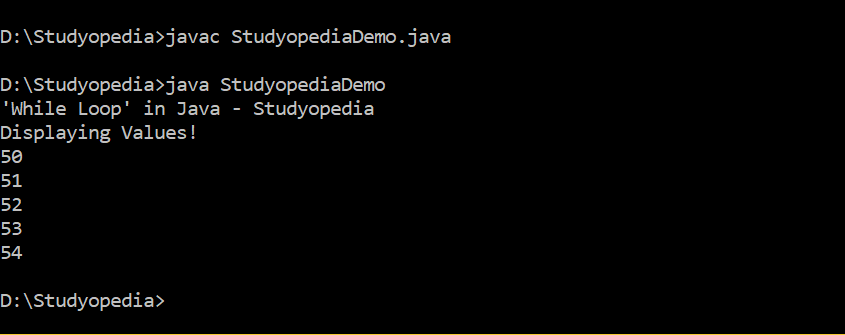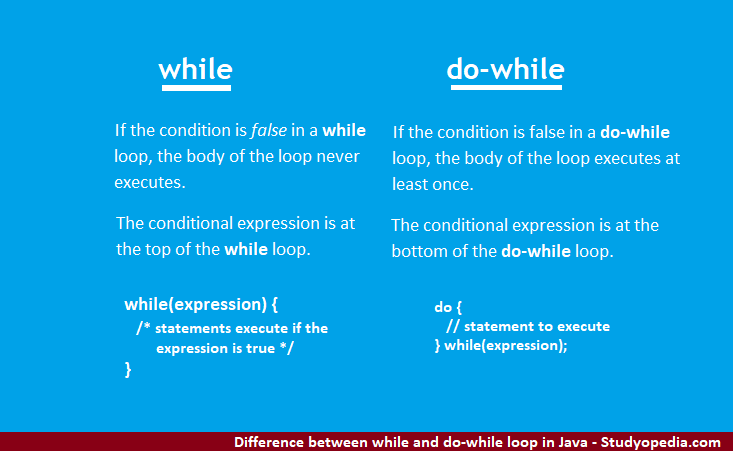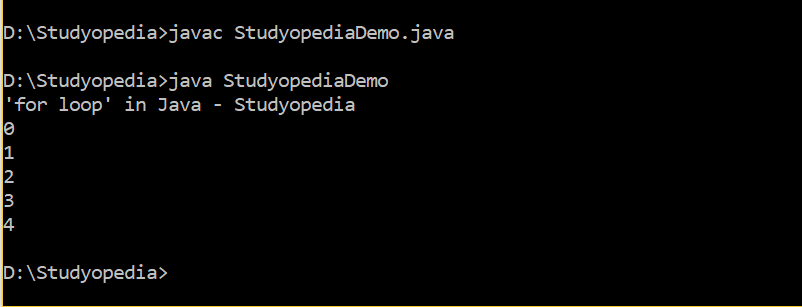## 08 May Loops in Java

Loops in Java, includes the following,

• while loop
• do-while loop
• for loop

Let us learn about the loops one by one,

## while loop

If the condition is true, a statement or a group of statements execute in while loop. As long as the condition/ expression is true, the statement in the body executes.

If the condition is false, the body of the loop will not execute.

### Syntax

Here, expression is a condition or a Boolean Expression,

### Example

Let us see an example, wherein we learn about the usage of while loop,

### Output

The following is the output,## do-while loop

The do-while loop executes at least once, even if the condition/ expression is false, unlike while loop.

If the condition is true, a statement or a group of statements execute in do-while loop

If the condition is false, the body of the loop executes at least once.

### Syntax

Here, expression is a boolean expression or condition,

### Example

Let us see an example, wherein we learn about the usage of do-while loop,

### Output

The following is the output,

## Difference between while and do-while loop

If the condition is false in a while loop, the body of the loop never executes.

If the condition is false in a do-while loop, the body of the loop executes at least once.

The conditional expression is at the top of the while loop.

The conditional expression is at the bottom of the do-while loop.Now let us learn about for loop in Java.

## for-loop

The for loop is a loop that executes a specified number of times. Under this, initialize, test, and increment loop counter in a single line, unlike while and do-while loop.

### Syntax

Here,

• initialization: declare and initialize any loop control variable
• expression: a Boolean expression if true, the statement is executed
• inc/ dec: increment/ decrement loop

### Example

Let us see an example, wherein we learn about the usage of for-loop,

### Output

The following is the output,In this lesson, we learned about loops in Java, while, do-while and for loop. In the next lesson we will learn about break and continue statements in Java.

##### Tags:[email protected]

We work to create programming tutorials for all.